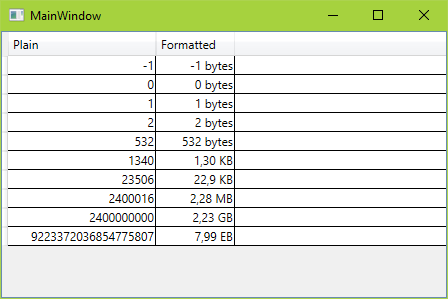# Presenting Byte Size Values in WPF

Though it's not my main job, I still enjoy writing WPF application. Small tools, making my colleagues' and my own life easier.

Recently I had the requirement to display size values in bytes, kilobytes, etc in a well-rounded way. You will find many examples for formatting such values in the internet. Most look like this:

``````string result;
if (number >= 1024 * 1024 * 1024) {
result = (number / 1024.0 / 1024 / 1024).ToString("F1") + " GB";
} else if (number >= 1024 * 1024) {
result = (number / 1024.0 / 1024).ToString("F1") + " MB";
} else if (number >= 1024) {
result = (number / 1024.0).ToString("F1") + " KB";
} else {
result = number + " Bytes";
}
``````

or, in a smarter way

``````string[] sizes = { "B", "KB", "MB", "GB", "TB" };
double len = number;
int order = 0;
while (len >= 1024 && order < sizes.Length - 1) {
order++;
len = len / 1024;
}
string result = \$"{len:0.##} {sizes[order]}";
``````

However, if you're on the Windows platform, there's a much easier option: `StrFormatByteSize`. That's the same method that Explorer is using to display file sizes. Its advantages are that you don't have any localization issues, and it it has a fixed precision of 3 digits.

Because my application is using WPF, I wrote a `IValueConverter` to be used in bindings:

Liquid error: One or more errors occurred.

Formatting binded values in XAML becomes quite easy with that converter (see full XAML):

``````<DataGrid
AutoGenerateColumns="False"
ItemsSource="{StaticResource numbers}">
<DataGrid.Resources>
<!--  the actual converter  -->
<local:FormatKbSizeConverter x:Key="FormatKbSizeConverter" />
</DataGrid.Resources>
<DataGrid.Columns>
<!--  First column shows the plain values  -->
<DataGridTextColumn
Binding="{Binding}"
ElementStyle="{StaticResource RightCell}"